Publicité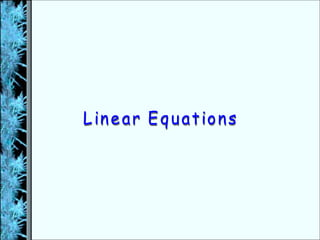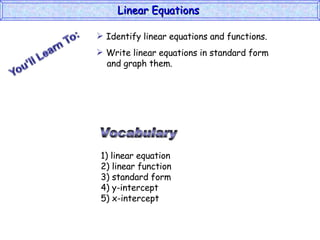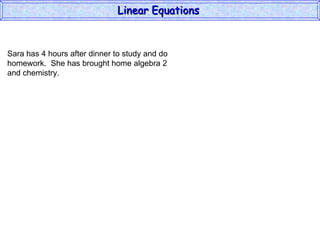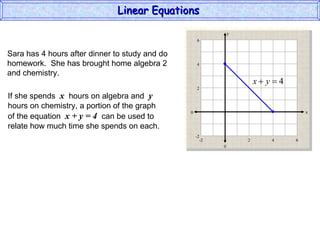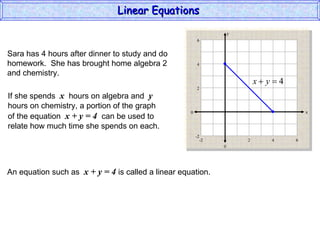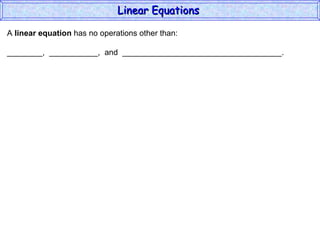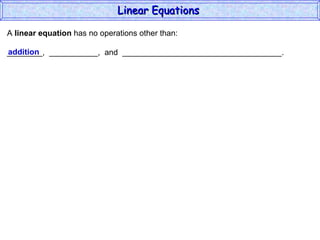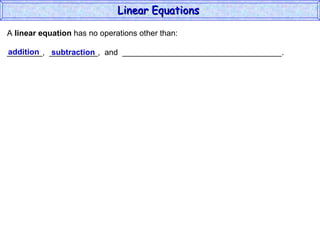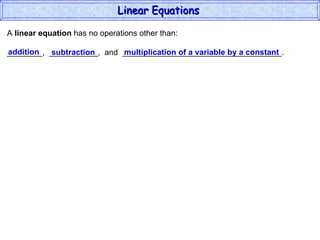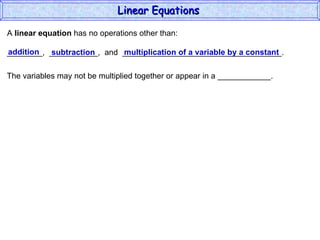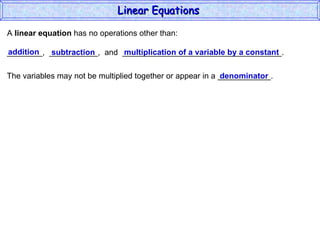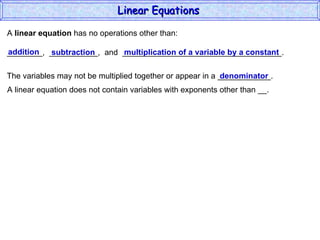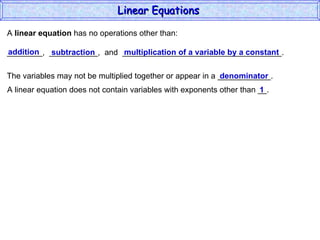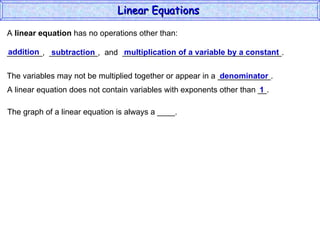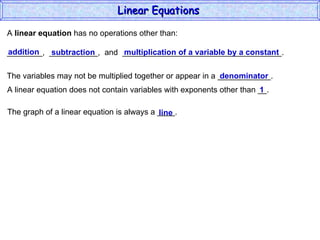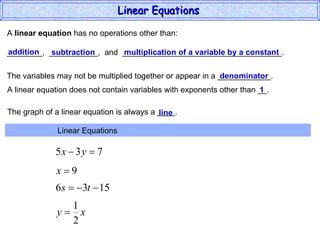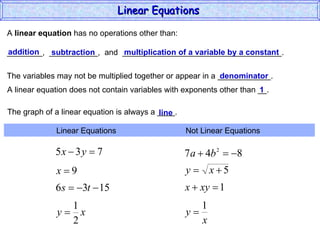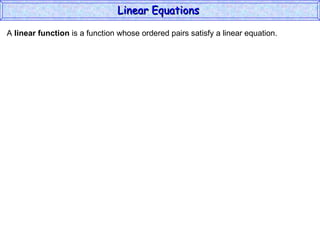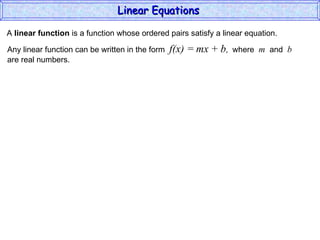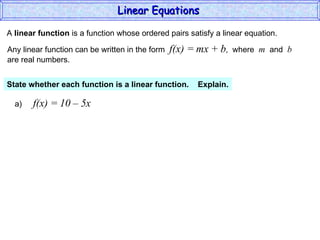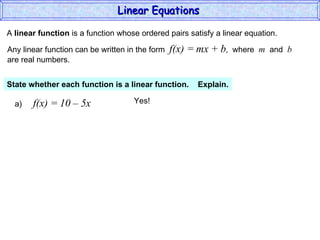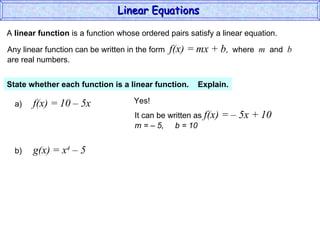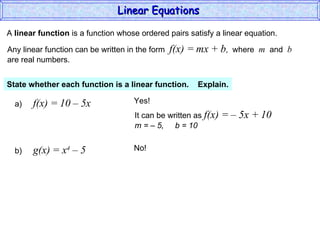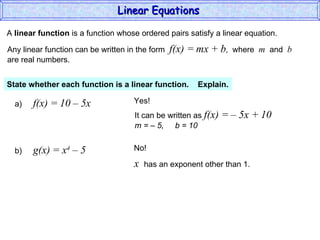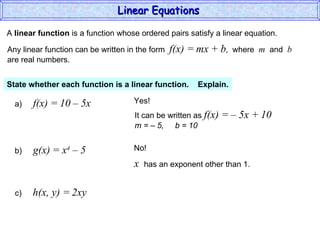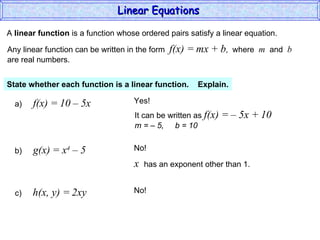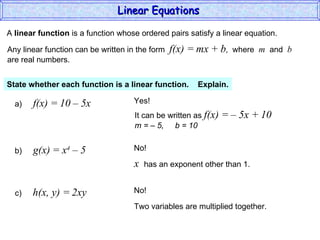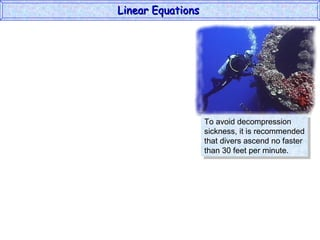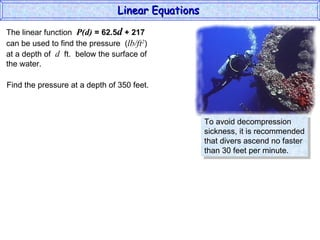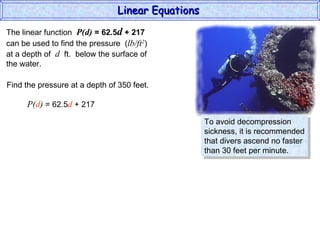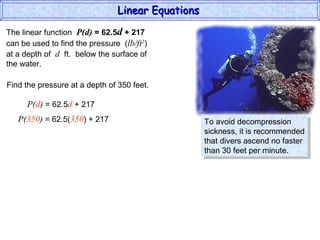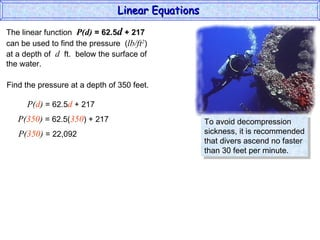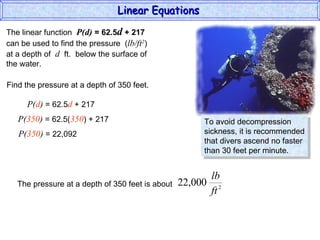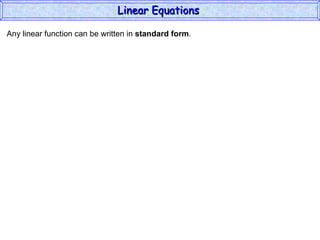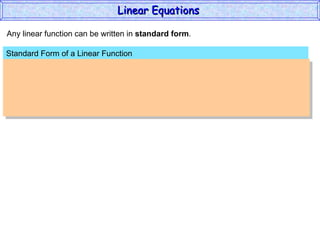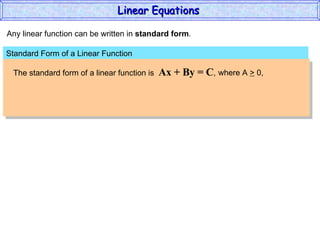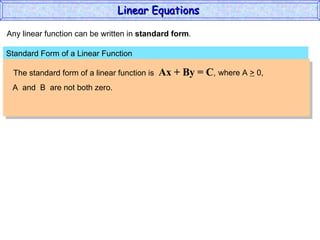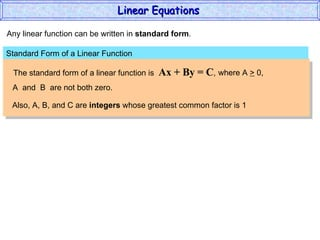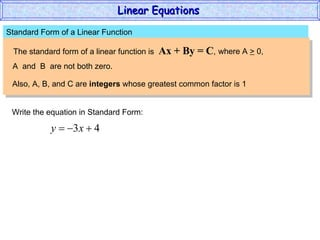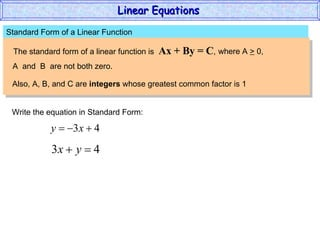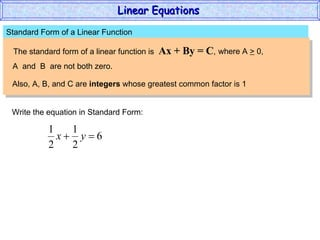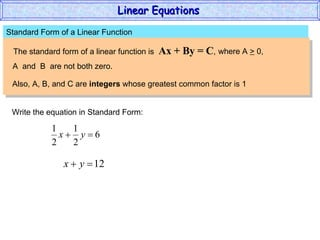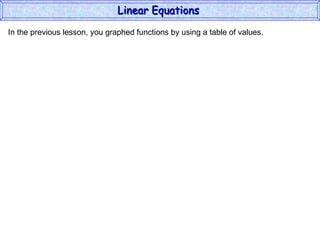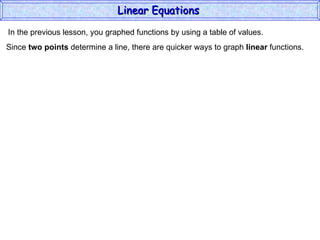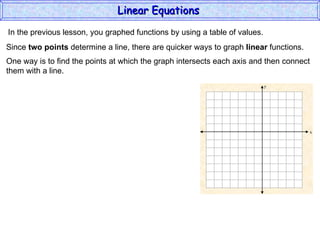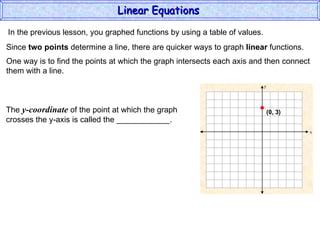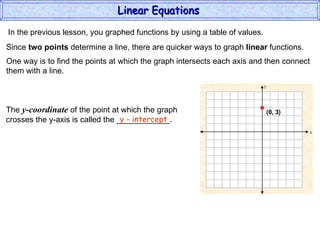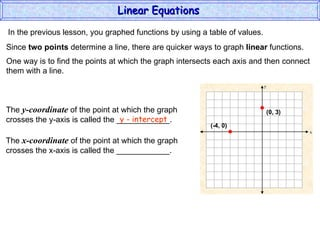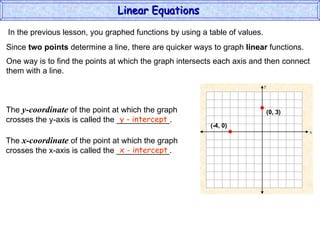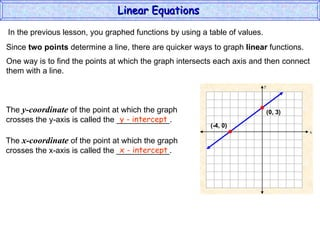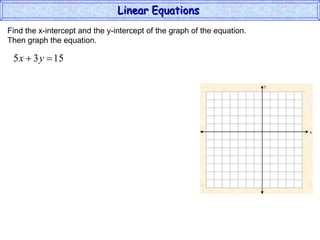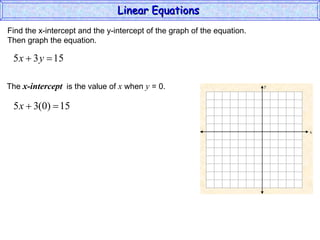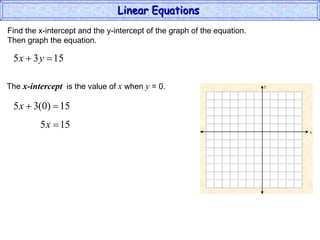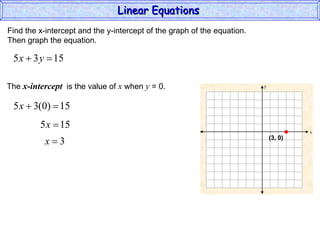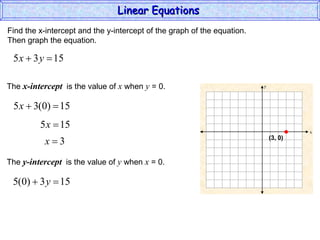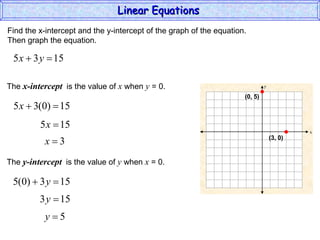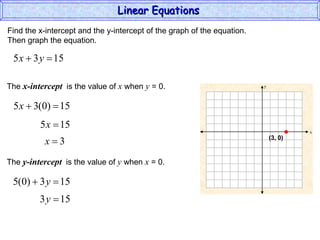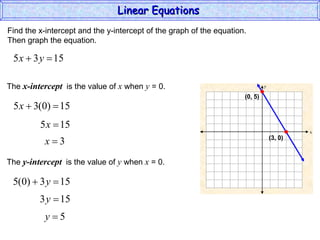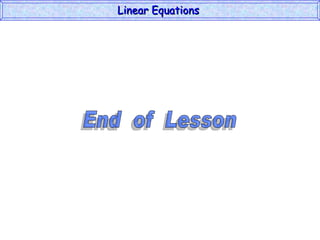1 sur 63
Publicité

### Linear Equations

1. Linear Equations
2. Sara has 4 hours after dinner to study and do homework. She has brought home algebra 2 and chemistry. Linear Equations
3. Sara has 4 hours after dinner to study and do homework. She has brought home algebra 2 and chemistry. If she spends x hours on algebra and y hours on chemistry, a portion of the graph of the equation x + y = 4 can be used to relate how much time she spends on each. Linear Equations y x 6 0 4 -2 -2 2 6 -2 6 0 -2 6 2 4
4. Sara has 4 hours after dinner to study and do homework. She has brought home algebra 2 and chemistry. If she spends x hours on algebra and y hours on chemistry, a portion of the graph of the equation x + y = 4 can be used to relate how much time she spends on each. An equation such as x + y = 4 is called a linear equation. Linear Equations y x 6 0 4 -2 -2 2 6 -2 6 0 -2 6 2 4
5. A linear equation has no operations other than: ________, ___________, and ____________________________________. Linear Equations
6. A linear equation has no operations other than: ________, ___________, and ____________________________________. addition Linear Equations
7. A linear equation has no operations other than: ________, ___________, and ____________________________________. addition subtraction Linear Equations
8. A linear equation has no operations other than: ________, ___________, and ____________________________________. addition subtraction multiplication of a variable by a constant Linear Equations
9. A linear equation has no operations other than: ________, ___________, and ____________________________________. addition subtraction multiplication of a variable by a constant The variables may not be multiplied together or appear in a ____________. Linear Equations
10. A linear equation has no operations other than: ________, ___________, and ____________________________________. addition subtraction multiplication of a variable by a constant The variables may not be multiplied together or appear in a ____________. denominator Linear Equations
11. A linear equation has no operations other than: ________, ___________, and ____________________________________. addition subtraction multiplication of a variable by a constant The variables may not be multiplied together or appear in a ____________. A linear equation does not contain variables with exponents other than __. denominator Linear Equations
12. A linear equation has no operations other than: ________, ___________, and ____________________________________. addition subtraction multiplication of a variable by a constant The variables may not be multiplied together or appear in a ____________. A linear equation does not contain variables with exponents other than __. 1 denominator Linear Equations
13. A linear equation has no operations other than: ________, ___________, and ____________________________________. addition subtraction multiplication of a variable by a constant The variables may not be multiplied together or appear in a ____________. A linear equation does not contain variables with exponents other than __. 1 denominator The graph of a linear equation is always a ____. Linear Equations
14. A linear equation has no operations other than: ________, ___________, and ____________________________________. addition subtraction multiplication of a variable by a constant The variables may not be multiplied together or appear in a ____________. A linear equation does not contain variables with exponents other than __. 1 denominator The graph of a linear equation is always a ____. line Linear Equations
15. A linear equation has no operations other than: ________, ___________, and ____________________________________. addition subtraction multiplication of a variable by a constant The variables may not be multiplied together or appear in a ____________. A linear equation does not contain variables with exponents other than __. 1 denominator The graph of a linear equation is always a ____. line Linear Equations Linear Equations
16. A linear equation has no operations other than: ________, ___________, and ____________________________________. addition subtraction multiplication of a variable by a constant The variables may not be multiplied together or appear in a ____________. A linear equation does not contain variables with exponents other than __. 1 denominator The graph of a linear equation is always a ____. line Linear Equations Not Linear Equations Linear Equations
17. A linear function is a function whose ordered pairs satisfy a linear equation. Linear Equations
18. A linear function is a function whose ordered pairs satisfy a linear equation. Any linear function can be written in the form f(x) = mx + b , where m and b are real numbers. Linear Equations
19. A linear function is a function whose ordered pairs satisfy a linear equation. Any linear function can be written in the form f(x) = mx + b , where m and b are real numbers. State whether each function is a linear function. Explain. a) f(x) = 10 – 5x Linear Equations
20. A linear function is a function whose ordered pairs satisfy a linear equation. Any linear function can be written in the form f(x) = mx + b , where m and b are real numbers. State whether each function is a linear function. Explain. a) f(x) = 10 – 5x Yes! Linear Equations
21. A linear function is a function whose ordered pairs satisfy a linear equation. Any linear function can be written in the form f(x) = mx + b , where m and b are real numbers. State whether each function is a linear function. Explain. a) f(x) = 10 – 5x Yes! It can be written as f(x) = – 5x + 10 m = – 5, b = 10 b) g(x) = x 4 – 5 Linear Equations
22. A linear function is a function whose ordered pairs satisfy a linear equation. Any linear function can be written in the form f(x) = mx + b , where m and b are real numbers. State whether each function is a linear function. Explain. a) f(x) = 10 – 5x Yes! It can be written as f(x) = – 5x + 10 m = – 5, b = 10 b) g(x) = x 4 – 5 No! Linear Equations
23. A linear function is a function whose ordered pairs satisfy a linear equation. Any linear function can be written in the form f(x) = mx + b , where m and b are real numbers. State whether each function is a linear function. Explain. a) f(x) = 10 – 5x Yes! It can be written as f(x) = – 5x + 10 m = – 5, b = 10 b) g(x) = x 4 – 5 No! x has an exponent other than 1. Linear Equations
24. A linear function is a function whose ordered pairs satisfy a linear equation. Any linear function can be written in the form f(x) = mx + b , where m and b are real numbers. State whether each function is a linear function. Explain. a) f(x) = 10 – 5x Yes! It can be written as f(x) = – 5x + 10 m = – 5, b = 10 b) g(x) = x 4 – 5 No! x has an exponent other than 1. c) h(x, y) = 2xy Linear Equations
25. A linear function is a function whose ordered pairs satisfy a linear equation. Any linear function can be written in the form f(x) = mx + b , where m and b are real numbers. State whether each function is a linear function. Explain. a) f(x) = 10 – 5x Yes! It can be written as f(x) = – 5x + 10 m = – 5, b = 10 b) g(x) = x 4 – 5 No! x has an exponent other than 1. c) h(x, y) = 2xy No! Linear Equations
26. A linear function is a function whose ordered pairs satisfy a linear equation. Any linear function can be written in the form f(x) = mx + b , where m and b are real numbers. State whether each function is a linear function. Explain. a) f(x) = 10 – 5x Yes! It can be written as f(x) = – 5x + 10 m = – 5, b = 10 b) g(x) = x 4 – 5 No! x has an exponent other than 1. c) h(x, y) = 2xy No! Two variables are multiplied together. Linear Equations
27. To avoid decompression sickness, it is recommended that divers ascend no faster than 30 feet per minute. Linear Equations
28. To avoid decompression sickness, it is recommended that divers ascend no faster than 30 feet per minute. The linear function P(d) = 62.5 d + 217 can be used to find the pressure ( lb/ft 2 ) at a depth of d ft. below the surface of the water. Linear Equations
29. To avoid decompression sickness, it is recommended that divers ascend no faster than 30 feet per minute. The linear function P(d) = 62.5 d + 217 can be used to find the pressure ( lb/ft 2 ) at a depth of d ft. below the surface of the water. Find the pressure at a depth of 350 feet. Linear Equations
30. To avoid decompression sickness, it is recommended that divers ascend no faster than 30 feet per minute. The linear function P(d) = 62.5 d + 217 can be used to find the pressure ( lb/ft 2 ) at a depth of d ft. below the surface of the water. Find the pressure at a depth of 350 feet. P( d ) = 62.5 d + 217 Linear Equations
31. To avoid decompression sickness, it is recommended that divers ascend no faster than 30 feet per minute. The linear function P(d) = 62.5 d + 217 can be used to find the pressure ( lb/ft 2 ) at a depth of d ft. below the surface of the water. Find the pressure at a depth of 350 feet. P( d ) = 62.5 d + 217 P( 350 ) = 62.5( 350 ) + 217 Linear Equations
32. To avoid decompression sickness, it is recommended that divers ascend no faster than 30 feet per minute. The linear function P(d) = 62.5 d + 217 can be used to find the pressure ( lb/ft 2 ) at a depth of d ft. below the surface of the water. Find the pressure at a depth of 350 feet. P( d ) = 62.5 d + 217 P( 350 ) = 62.5( 350 ) + 217 P( 350 ) = 22,092 Linear Equations
33. To avoid decompression sickness, it is recommended that divers ascend no faster than 30 feet per minute. The linear function P(d) = 62.5 d + 217 can be used to find the pressure ( lb/ft 2 ) at a depth of d ft. below the surface of the water. Find the pressure at a depth of 350 feet. P( d ) = 62.5 d + 217 P( 350 ) = 62.5( 350 ) + 217 P( 350 ) = 22,092 Linear Equations The pressure at a depth of 350 feet is about
34. Any linear function can be written in standard form . Linear Equations
35. Any linear function can be written in standard form . Linear Equations Standard Form of a Linear Function
36. Any linear function can be written in standard form . The standard form of a linear function is Ax + By = C , where A > 0, Linear Equations Standard Form of a Linear Function
37. Any linear function can be written in standard form . The standard form of a linear function is Ax + By = C , where A > 0, A and B are not both zero. Linear Equations Standard Form of a Linear Function
38. Any linear function can be written in standard form . The standard form of a linear function is Ax + By = C , where A > 0, A and B are not both zero. Also, A, B, and C are integers whose greatest common factor is 1 Linear Equations Standard Form of a Linear Function
39. The standard form of a linear function is Ax + By = C , where A > 0, A and B are not both zero. Also, A, B, and C are integers whose greatest common factor is 1 Linear Equations Write the equation in Standard Form: Standard Form of a Linear Function
40. The standard form of a linear function is Ax + By = C , where A > 0, A and B are not both zero. Also, A, B, and C are integers whose greatest common factor is 1 Linear Equations Write the equation in Standard Form: Standard Form of a Linear Function
41. The standard form of a linear function is Ax + By = C , where A > 0, A and B are not both zero. Also, A, B, and C are integers whose greatest common factor is 1 Linear Equations Write the equation in Standard Form: Standard Form of a Linear Function
42. The standard form of a linear function is Ax + By = C , where A > 0, A and B are not both zero. Also, A, B, and C are integers whose greatest common factor is 1 Linear Equations Write the equation in Standard Form: Standard Form of a Linear Function
43. In the previous lesson, you graphed functions by using a table of values. Linear Equations
44. In the previous lesson, you graphed functions by using a table of values. Since two points determine a line, there are quicker ways to graph linear functions. Linear Equations
45. In the previous lesson, you graphed functions by using a table of values. Since two points determine a line, there are quicker ways to graph linear functions. One way is to find the points at which the graph intersects each axis and then connect them with a line. Linear Equations y x
46. In the previous lesson, you graphed functions by using a table of values. Since two points determine a line, there are quicker ways to graph linear functions. One way is to find the points at which the graph intersects each axis and then connect them with a line. The y-coordinate of the point at which the graph crosses the y-axis is called the ____________. Linear Equations y x (0, 3)
47. In the previous lesson, you graphed functions by using a table of values. Since two points determine a line, there are quicker ways to graph linear functions. One way is to find the points at which the graph intersects each axis and then connect them with a line. The y-coordinate of the point at which the graph crosses the y-axis is called the ____________. y - intercept Linear Equations y x (0, 3)
48. In the previous lesson, you graphed functions by using a table of values. Since two points determine a line, there are quicker ways to graph linear functions. One way is to find the points at which the graph intersects each axis and then connect them with a line. The y-coordinate of the point at which the graph crosses the y-axis is called the ____________. y - intercept The x-coordinate of the point at which the graph crosses the x-axis is called the ____________. Linear Equations y x (0, 3) (-4, 0)
49. In the previous lesson, you graphed functions by using a table of values. Since two points determine a line, there are quicker ways to graph linear functions. One way is to find the points at which the graph intersects each axis and then connect them with a line. The y-coordinate of the point at which the graph crosses the y-axis is called the ____________. y - intercept The x-coordinate of the point at which the graph crosses the x-axis is called the ____________. x - intercept Linear Equations y x (0, 3) (-4, 0)
50. In the previous lesson, you graphed functions by using a table of values. Since two points determine a line, there are quicker ways to graph linear functions. One way is to find the points at which the graph intersects each axis and then connect them with a line. The y-coordinate of the point at which the graph crosses the y-axis is called the ____________. y - intercept The x-coordinate of the point at which the graph crosses the x-axis is called the ____________. x - intercept Linear Equations y x (0, 3) (-4, 0)
51. Find the x-intercept and the y-intercept of the graph of the equation. Then graph the equation. Linear Equations y x
52. Find the x-intercept and the y-intercept of the graph of the equation. Then graph the equation. The x-intercept is the value of x when y = 0. Linear Equations y x
53. Find the x-intercept and the y-intercept of the graph of the equation. Then graph the equation. The x-intercept is the value of x when y = 0. Linear Equations y x
54. Find the x-intercept and the y-intercept of the graph of the equation. Then graph the equation. The x-intercept is the value of x when y = 0. Linear Equations y x (3, 0)
55. Find the x-intercept and the y-intercept of the graph of the equation. Then graph the equation. The x-intercept is the value of x when y = 0. Linear Equations The y-intercept is the value of y when x = 0. y x (3, 0)
56. Find the x-intercept and the y-intercept of the graph of the equation. Then graph the equation. The x-intercept is the value of x when y = 0. Linear Equations The y-intercept is the value of y when x = 0. y x (0, 5) (3, 0)
57. Find the x-intercept and the y-intercept of the graph of the equation. Then graph the equation. The x-intercept is the value of x when y = 0. Linear Equations The y-intercept is the value of y when x = 0. y x (0, 5) (3, 0)
58. Find the x-intercept and the y-intercept of the graph of the equation. Then graph the equation. The x-intercept is the value of x when y = 0. Linear Equations The y-intercept is the value of y when x = 0. y x (3, 0)
59. Find the x-intercept and the y-intercept of the graph of the equation. Then graph the equation. The x-intercept is the value of x when y = 0. Linear Equations The y-intercept is the value of y when x = 0. y x (3, 0) (0, 5)
60. Find the x-intercept and the y-intercept of the graph of the equation. Then graph the equation. The x-intercept is the value of x when y = 0. Linear Equations The y-intercept is the value of y when x = 0. y x (0, 5) (3, 0)
61. End of Lesson Linear Equations
62. Credits PowerPoint created by Using Glencoe’s Algebra 2 text, © 2005 Robert Fant http://robertfant.com
Publicité